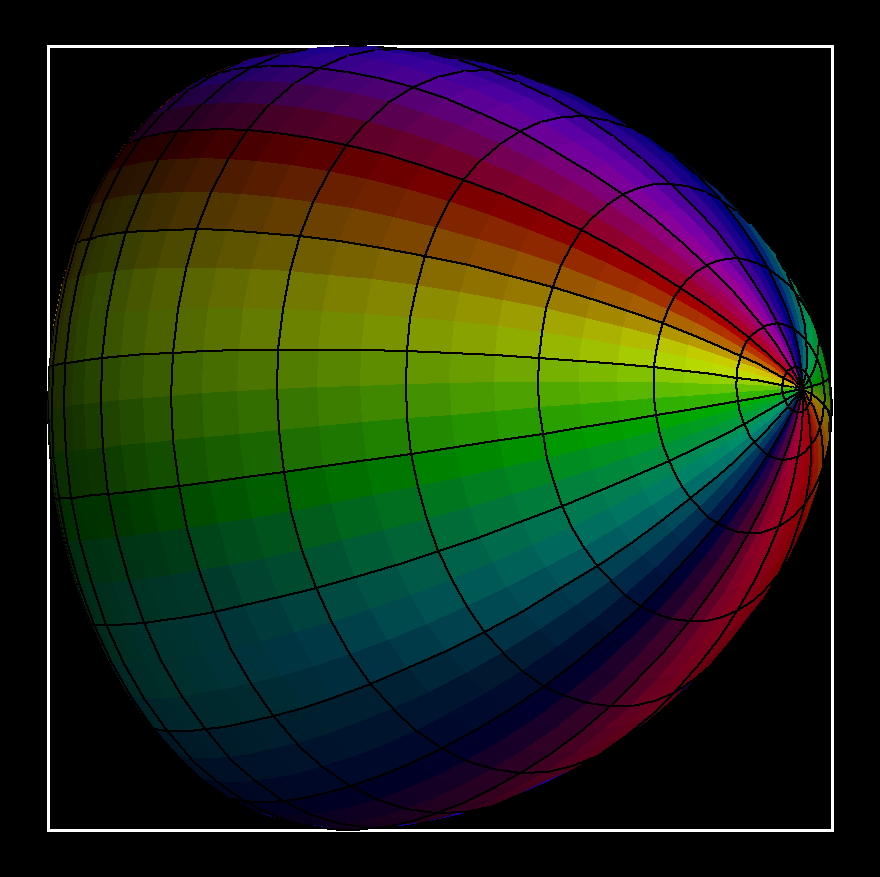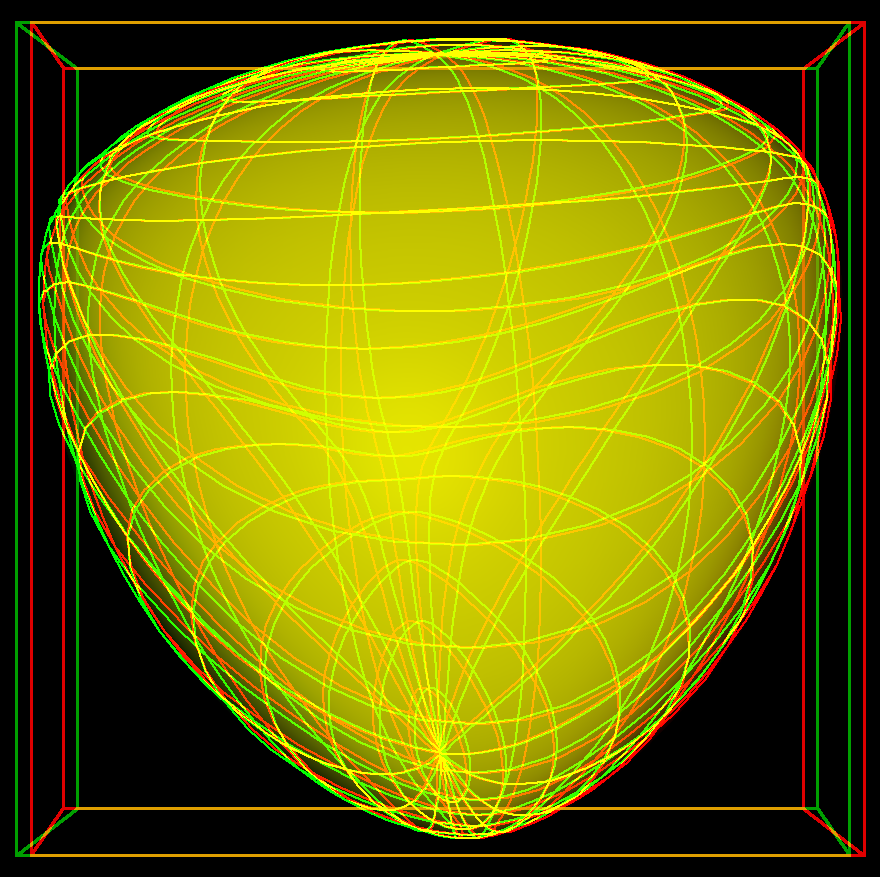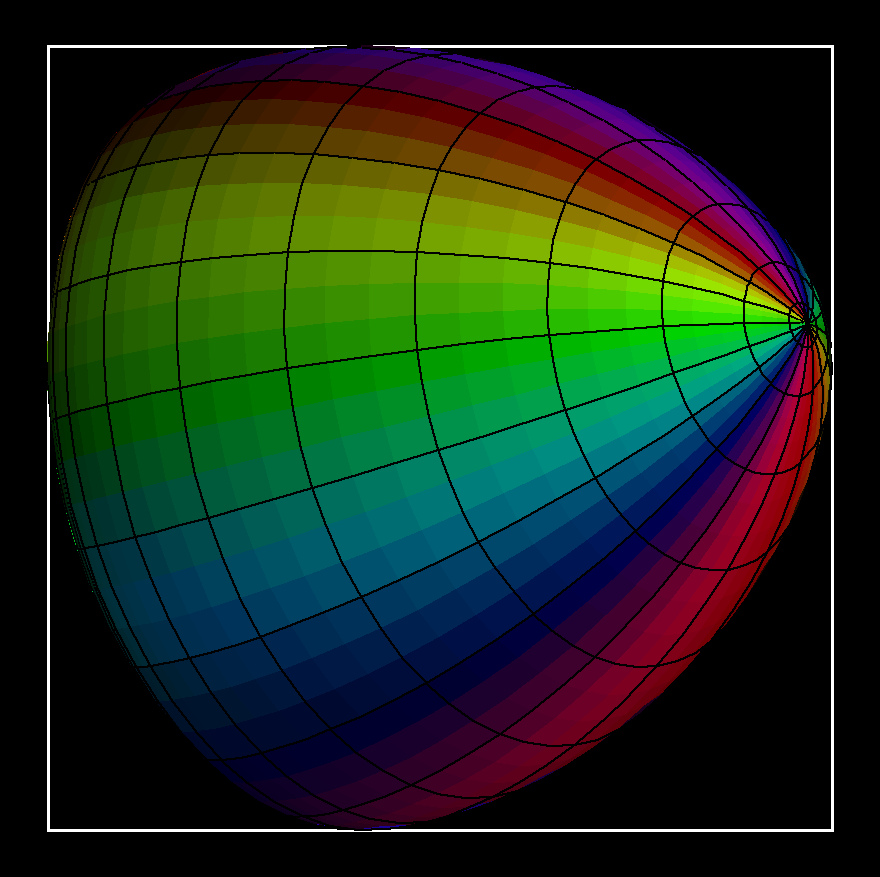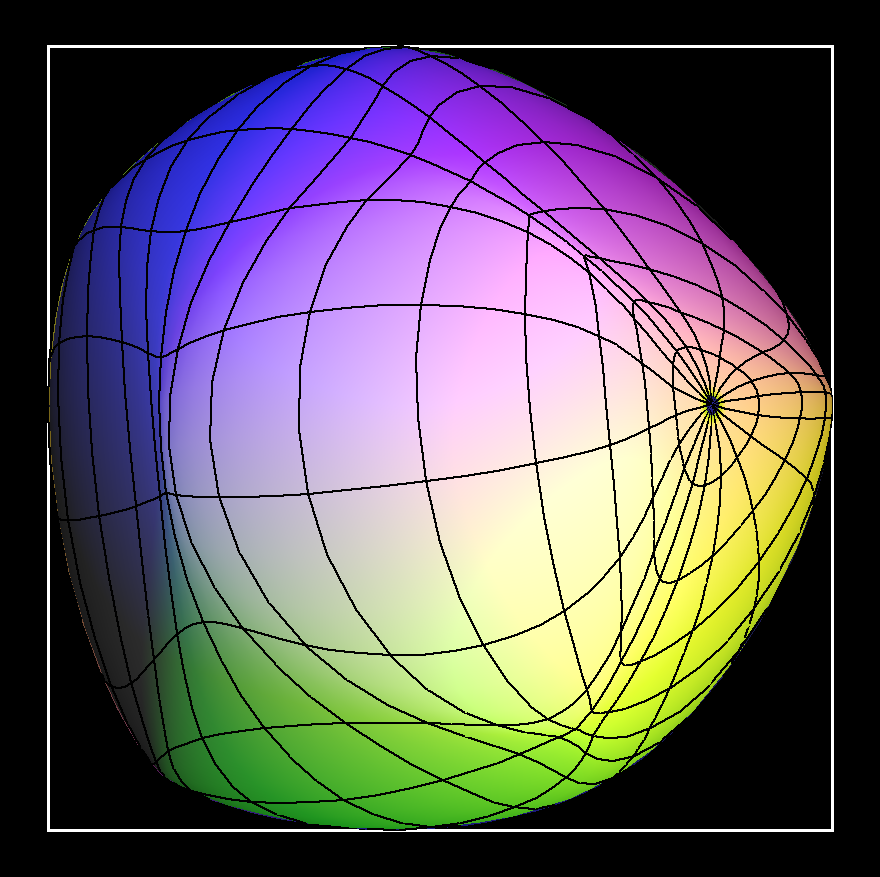# Surfaces of Constant Width

A convex surface which has in every direction the same diameter is called “surface of constant width”. The diameter in any given direction is the distance between the two (opposite) tangent planes orthogonal to that direction.Take a Convex Curves of constant width which has a symmetry axis. Rotation around the axis gives a (rotationally symmetric) surface of constant width.

Surfaces of constant width can move around in a cubical box which they touch at all six faces. This “rotation” is more a wobbling around since no “midpoint” stays fixed.A more complicated surface of constant width "rotates" in its box.One can morph the above two surfaces into each other through surfaces of constant width.A still more complicated surface of constant width rotating in its box.A surface of constant width with diehedral symmetr D3 is morphed through surfaces of constant width into the previous surface.

## Explicit Formulas

A convex surface, given as the image of a map F: S^2 ⟶ R^3, can be defined by its "support function" h : S^2 ⟶ R+ by putting for each vector n in S^2

`F(n) = h(n) * n + grad h(n) `

(grad h understood as tangent vector of S^2)

The vector n is the exterior normal of the surface at the point F(n).

The image of F is a surface of constant width w, iff `h(n) + h(-n) = w`.

If we write

`n = (x,y,z) with x^2 + y^2 + z^2 = 1`

, then we take

`h(x,y,z) = a + b*z^3 + c*xy^2 + d*yz^2 + e*xz^2 + f*xyz + g*xy^2z^2`

The first constant a has to be large enough so that the values of h are positive.

Get red/blue stereo glasses from amazon

Constant_Width.pdf